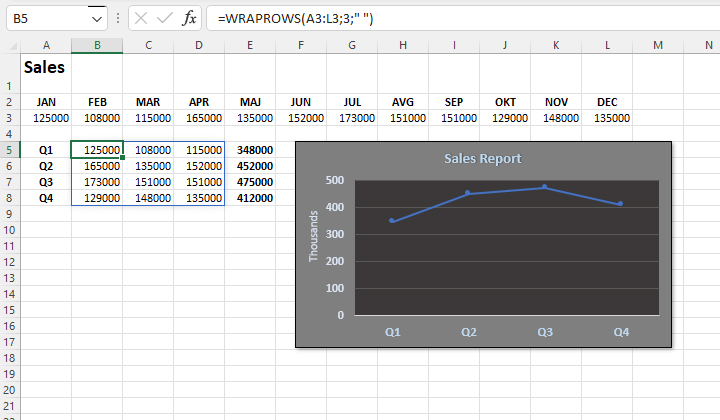# Wraping reports

When creating a report, there will sometimes be a need to rearrange rows and/or columns into a form suitable for reporting. Previously, you had to do this manually, and now there are two functions that can make the said job easier for you. They are named WRAPROWS and WRAPCOLUMNS. Let’s see how you can “wrap” the data in a way that suits you.

The syntax of the WRAPROWS function is:

WRAPROWS (<string>, <number of rows>, <replace value>)

The first argument of this function is an array- the vector you want to parse. The second argument is the number of columns that will be occupied by the newly formed matrix, and the last one represents the value with which the function will replace all those cells where it returns an empty result, that is an N/A error. If you specify a blank character here, as with the TOROW and TOCOL functions, the error will be replaced by the given symbol.

If we have a table that contains one row, which is the sales values by month, and we want to get the sales values by quarters, the easiest way to achieve this is by entering the formula:

=WRAPROWS(A3:L3,3,” “)

One row containing 12 cells is divided into 3 rows by 4 columns. Each newly created row shows sales in one quarter. If we calculate total we can easily create a chart that shows sales by quaters.

The WRAPCOLUMNS function works in the same way, except that it transforms columns into rows. Its syntax is:

WRAPCOLUMNS (<string>, <number of columns>, <replace value>)